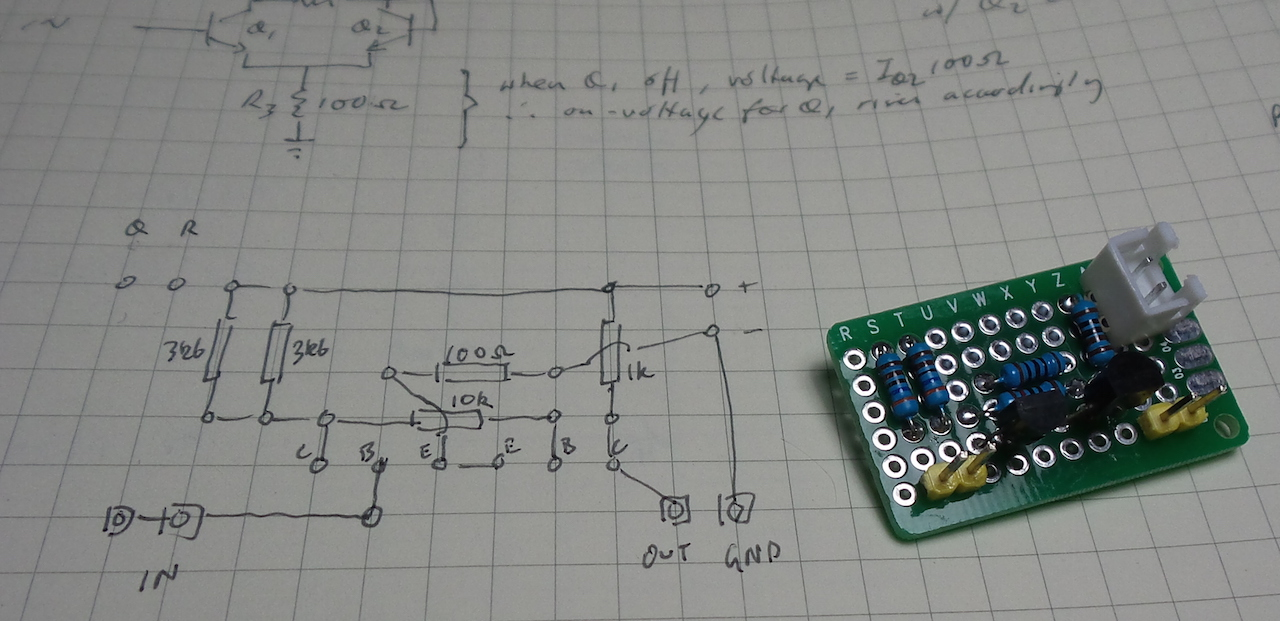# #317 Basic Discrete Schmitt Trigger

Exploring a basic emitter-coupled Schmitt Trigger circuit design with discrete components.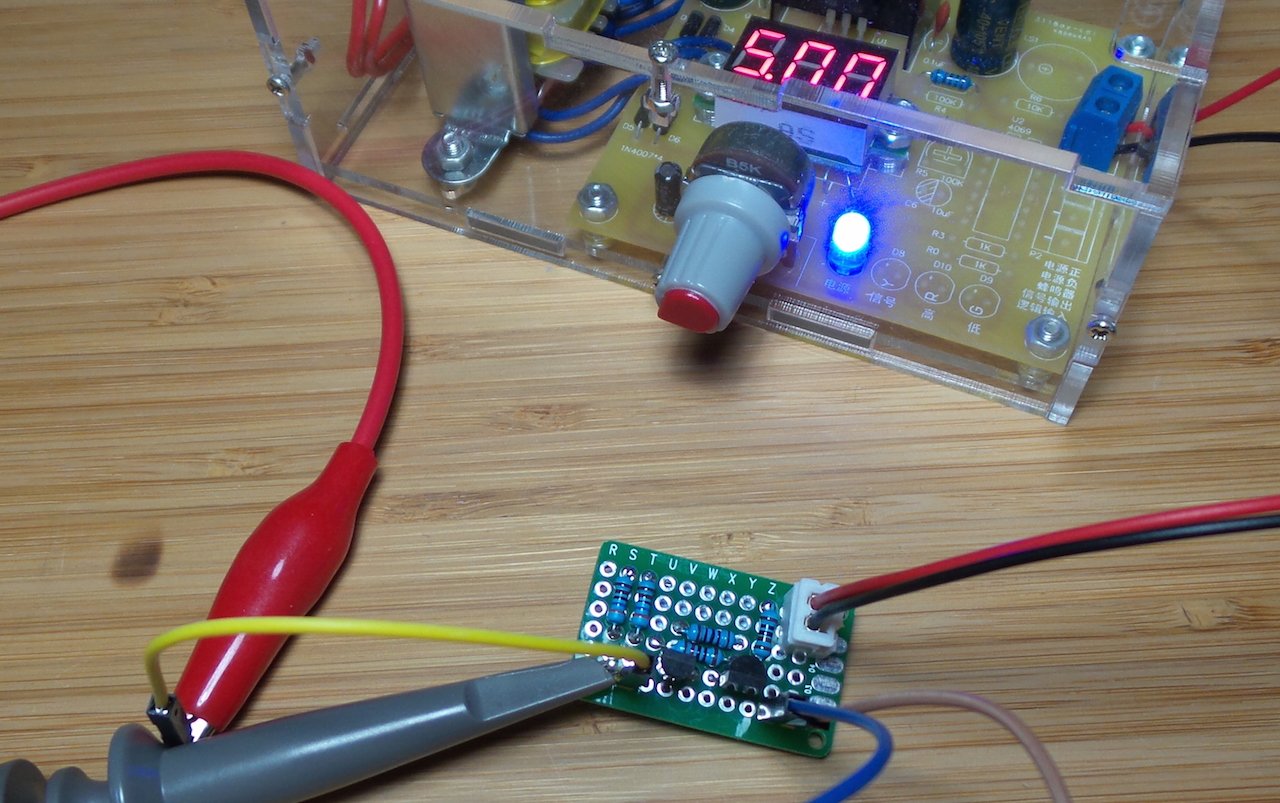A Schmitt trigger is a comparator circuit with hysteresis such that:

• the output swings high when the input rises above an upper threshold
• the output swings low when the input rises above a lower threshold

When upper threshold > lower threshold, the resulting hysteresis negates the impact of noise or minor oscillations on the input signal.

Schmitt triggers are commonly built into the input pins of integrated circuits to provide more definitive switching. Stand-alone Schmitt triggers are often constructed from comparators, but the most basic Schmitt trigger can be implemented with discrete components. This is described in the Art of Electronics (Second Edition p232).

This project explores the classic emitter-coupled Schmitt Trigger circuit design.

### Emitter-coupled Schmitt Trigger Operation

When Q1 collector resistor R1 is greater than the Q2 collector resistor R2, then the voltage drop over the common emitter resistor R3 is higher when Q2 is on.

The threshold to tun Q1 on or off depends on the voltage drop over the R3.

Thus:

• when input is low:
• Q1 is off, Q2 is on
• output is “low”
• and the “on” threshold for Q1 is pushed up by the higher R3 voltage drop
• when input is high:
• Q1 is on, Q2 is off
• output is “high”
• and the “off” threshold for Q1 is reduced by the lower R3 voltage drop

In other words, the hysteresis between upper (on) and lower (off) thresholds is defined by the differential R3 voltage drop.

### Practical Measurements

With a 1kHz input sine wave (CH1) the output (CH2) switches with hysteresis:

• swings high at ~1.3V
• swings low at ~0.9V
• so ~0.4V differential between upper and lower thresholds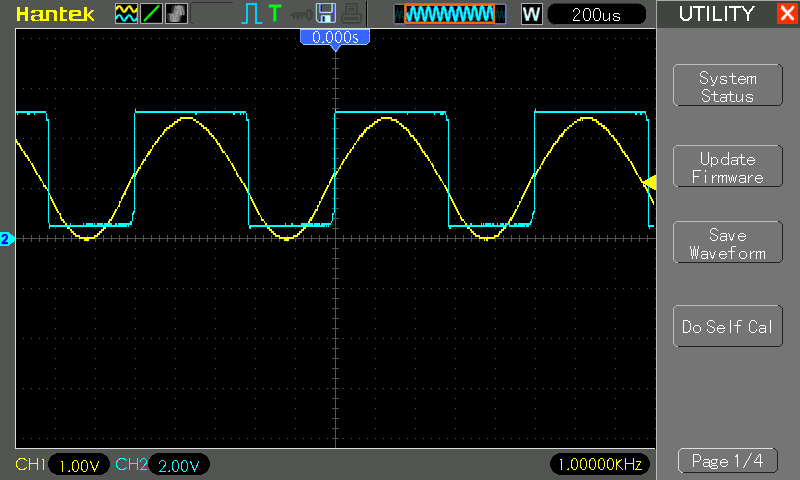An X-Y plot (X: input, Y: output voltage) captures the ~ 0.4V differential between the upper and lower thresholds.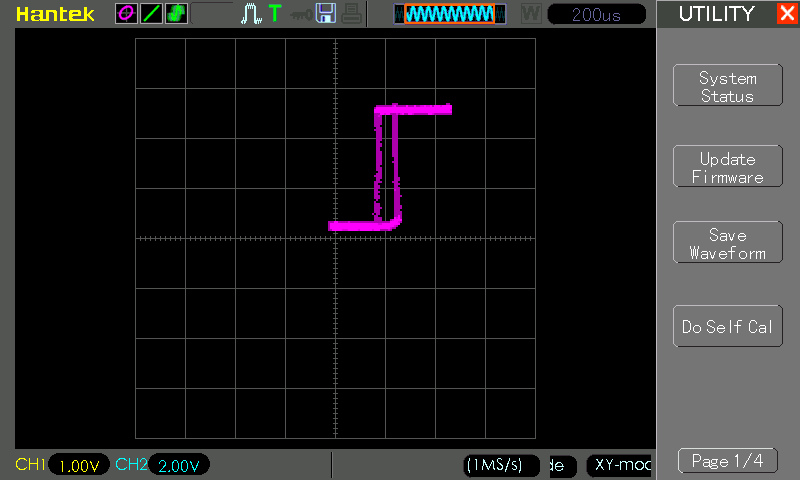At higher frequencies (here at 100kHz), the behaviour is impaired with some lag:## Construction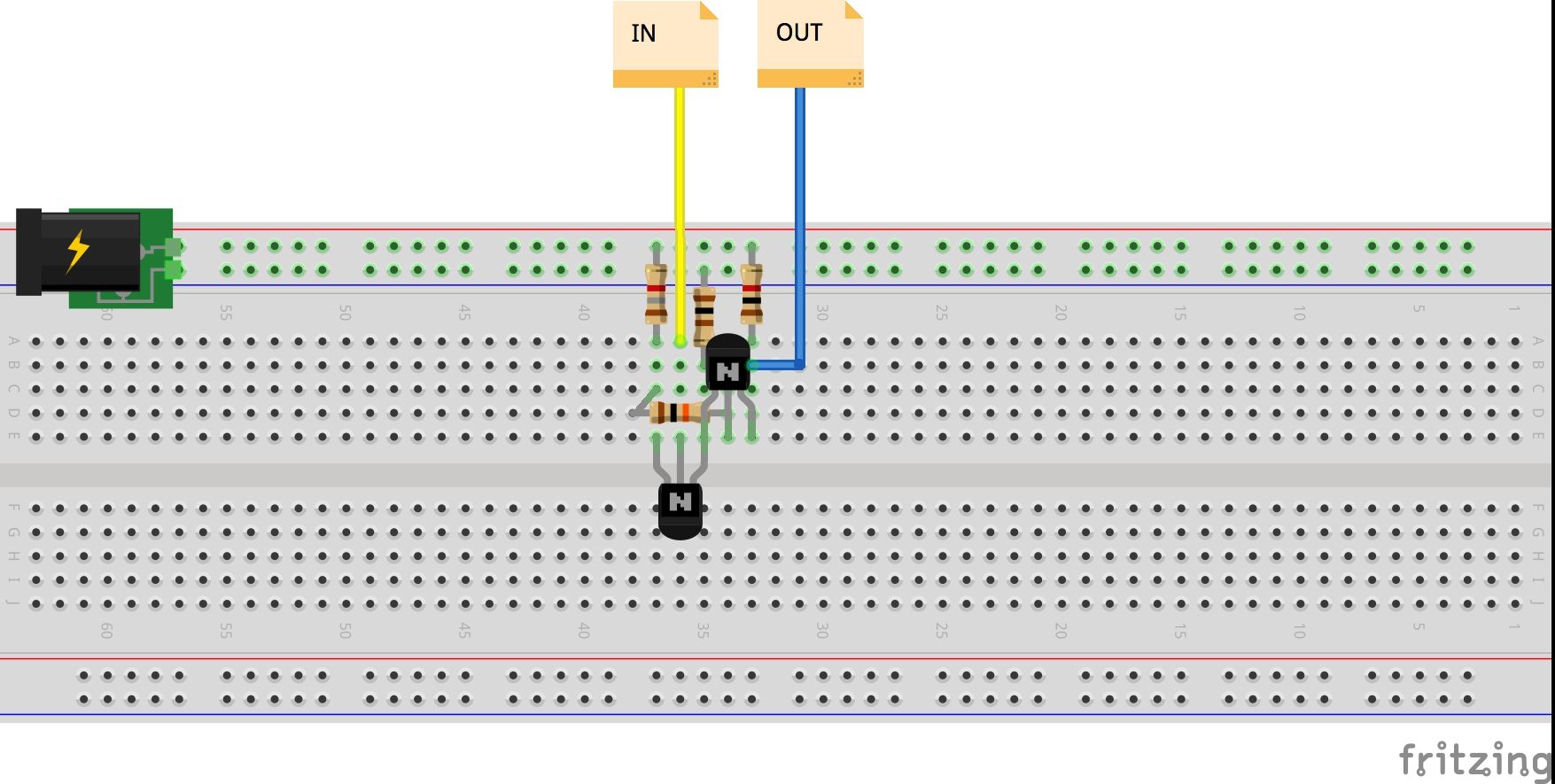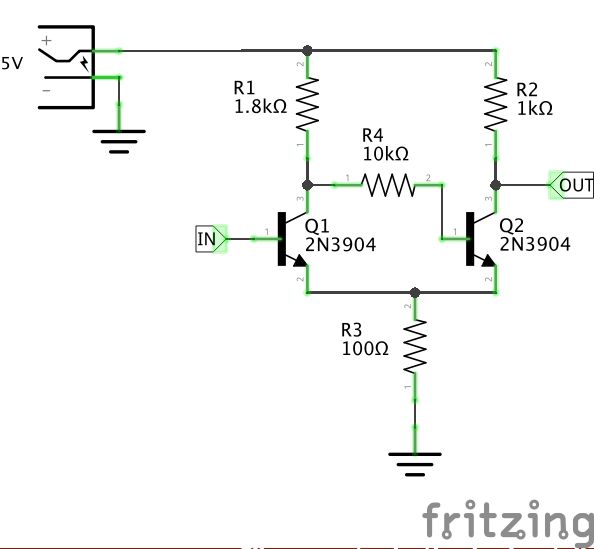Testing the circuit on a breadboard. Note I’m using 2 x 3.6kΩ resistors in parallel for R1.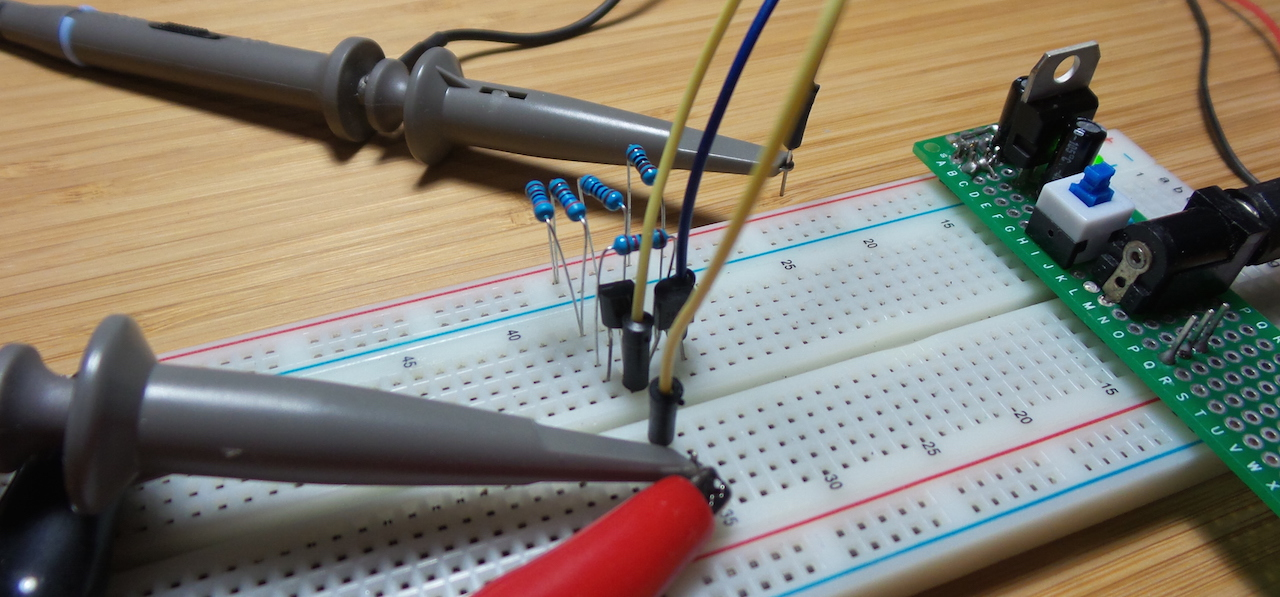Arranged and put on a protoboard for final tests: